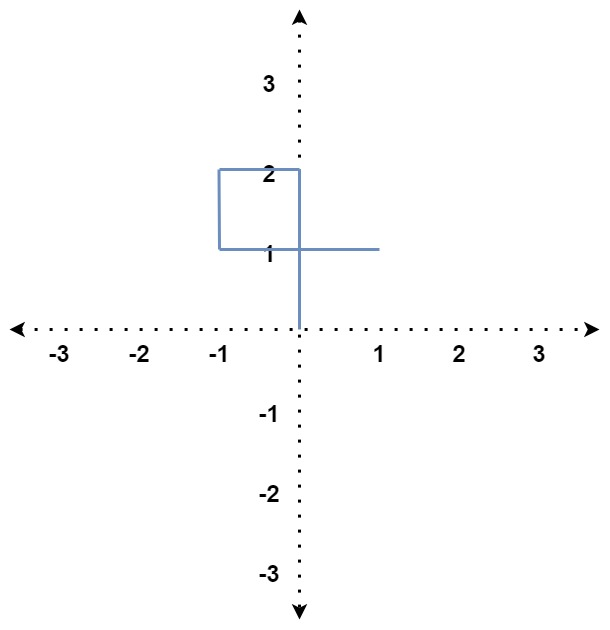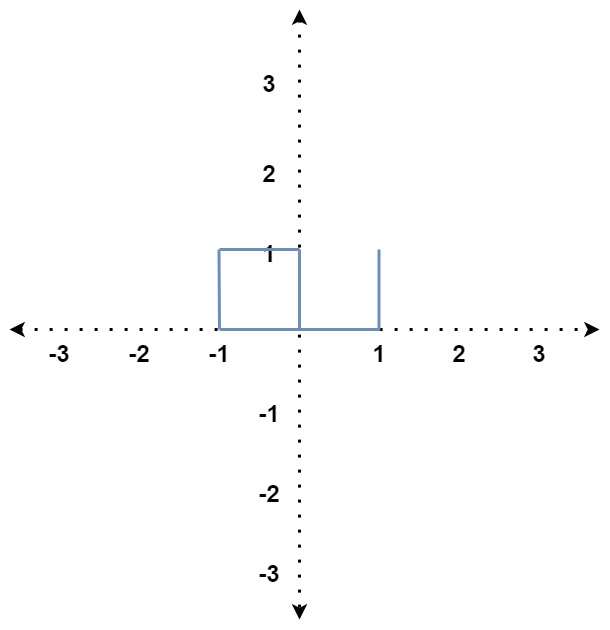## Algorithm

Problem Name: 335. Self Crossing

You are given an array of integers `distance`.

You start at the point `(0, 0)` on an X-Y plane, and you move `distance` meters to the north, then `distance` meters to the west, `distance` meters to the south, `distance` meters to the east, and so on. In other words, after each move, your direction changes counter-clockwise.

Return `true` if your path crosses itself or `false` if it does not.

Example 1:```Input: distance = [2,1,1,2]
Output: true
Explanation: The path crosses itself at the point (0, 1).
```

Example 2:```Input: distance = [1,2,3,4]
Output: false
Explanation: The path does not cross itself at any point.
```

Example 3:```Input: distance = [1,1,1,2,1]
Output: true
Explanation: The path crosses itself at the point (0, 0).
```

Constraints:

• `1 <= distance.length <= 105`
• `1 <= distance[i] <= 105`

## Code Examples

### #1 Code Example with Javascript Programming

```Code - Javascript Programming```

``````
const isSelfCrossing = function(x) {
for (let i = 3, l = x.length; i < l; i++) {
// Case 1: current line crosses the line 3 steps ahead of it
if (x[i] >= x[i - 2] && x[i - 1] <= x[i - 3]) return true
// Case 2: current line crosses the line 4 steps ahead of it
else if (i >= 4 && x[i - 1] == x[i - 3] && x[i] + x[i - 4] >= x[i - 2])
return true
// Case 3: current line crosses the line 6 steps ahead of it
else if (
i >= 5 &&
x[i - 2] >= x[i - 4] &&
x[i] + x[i - 4] >= x[i - 2] &&
x[i - 1] <= x[i - 3] &&
x[i - 1] + x[i - 5] >= x[i - 3]
)
return true
}
return false
}
``````
Copy The Code &

Input

cmd
distance = [2,1,1,2]

Output

cmd
true

### #2 Code Example with Python Programming

```Code - Python Programming```

``````
class Solution:
def isSelfCrossing(self, x: List[int]) -> bool:
b = c = d = e = 0
for a in x:
if d >= b > 0 and (a >= c or a >= c-e >= 0 and f >= d-b):
return True
b, c, d, e, f = a, b, c, d, e
return False
``````
Copy The Code &

Input

cmd
distance = [1,2,3,4]

Output

cmd
false